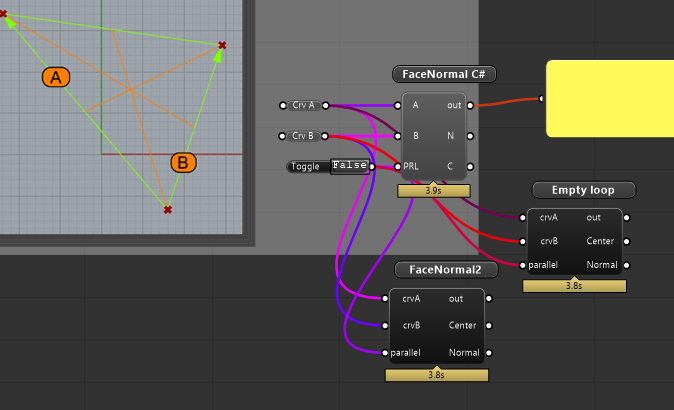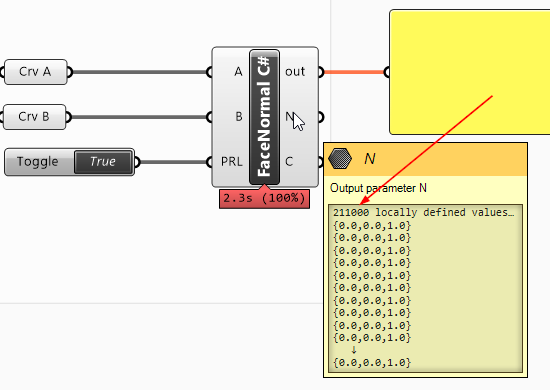# Speed Contest - Triangle Normal And Center

OK, so i’m trying to find the fastest way to calculate Face Normals and Face Center in my own C# code, but so far I’m not impressed by my achievements.

Edit: Ops. forgot the gh definition: TriNormalAndCenter.gh (17.4 KB)

Fig 1. Processing triangle legs “A” and “B” some 210.000 times:

The picture above is manually drawn, and the processing time is all internal, and the resulting normals and center points were output into two arrays (N and C)

The essential code I’ve tried.

``````    // =======
// NORMALS (CROSS PRODUCT)
// =======
// Right hand rule of lines a\/b
m_normals[i] = Vector3d.CrossProduct(line_b.Direction, line_a.Direction);
m_normals[i].Unitize();

// =======
// CENTER
// =======
var mida = line_a.PointAt(0.5);
var midb = line_b.PointAt(0.5);
// Criss-cross lines for intersection point at triangle center
var mida_c = (new Line(mida, line_b.To));
var midb_b = (new Line(midb, line_a.To));
// Line-Line Intersect
var _a = double.MinValue;
var _b = double.MinValue;
if (!Rhino.Geometry.Intersect.Intersection.LineLine(mida_c, midb_b, out _a, out _b))
return false;
m_centers[i] = mida_c.PointAt(_a);
``````

(Far below some “manual” equivalents to Rhino’s `Vector3d.CrossProduct` and `Line.PointAt(t)` but that didn’t make much of a difference either).

The parallel loop was partitioned so as to not cause extra overhead for multiple small operations

``````    if (parallel)
{
var partitioner = System.Collections.Concurrent.Partitioner.Create(0, count);
{
// Loop over each range element
for (int j = range.Item1; j < range.Item2; j++)
{
NormalAndCenterFromLines(j, line_a, line_b);
}
});
}
``````

Placing the code meat inline in the loop didn’t make much of a difference. Also, since there are only three inputs and the loop just repeats itself, it shouldn’t matter much that this was tested in a C# ScriptComponent instead of VS component. Is it the Line-Line intersetion test which consumes all this processing time?

Anyway, the final solution is meant to traverse Mesh edges and do the same (Rhino Mesh of course has methods for all this, but I need to do some tweaks and also make my own classes or structs for some of my mesh manipulations).

Anyone?

// Rolf

Addendum: “Manual” equivalents to Rhino’s `Vector3d.CrossProduct` and `Line.PointAt(t)`

``````    // =======
// NORMALS (CROSS PRODUCT)
// =======
// Right hand rule for lines a\/b
var a = line_b.Direction;
var b = line_a.Direction;
m_normals[i].X = (a.Y * b.Z) - (a.Z * b.Y);
m_normals[i].Y = (a.Z * b.X) - (a.X * b.Z);
m_normals[i].Z = (a.X * b.Y) - (a.Y * b.X);
m_normals[i].Unitize();
``````

and `Line.PointAt(t)`

``````    // "point on line" in Vector form
// (x,y,z) = (x0,y0,z0) + t(a,b,c)
var mid_a = line_a.From + 0.5 * line_a.Direction;
var mid_b = line_b.From + 0.5 * line_b.Direction;
``````

you can calculate the face Center by averaging all 3 (or 4) points. Simple add all vertices and divide by vertex count. To get the centernormal you calculate the vector of pt0 and ptC and pt1 and ptC and take the Cross of both.

Edit: I think Rhinocommon doesn’t allow you to add points (this usually doesn’t make sense), so just take each coordinate and add and divide them.

You can do that: Point3d TheLord = (The + Lord)/2.0; (where the 2 are Point3d).

1M Q: is any money involved in that contest? Vodka? cigars? something?

1 Like

A free link to a picture of a Ducati?// Rolf

1 Like

Try: a derestricted Termi for a Panigale V4S, a Rapid Bike Evo pro (+ valid maps) for a 1299S, a full carbon fairing for a 998S … or a 666 page manual about how to start your Ducati when it rains.BTW: Given p1, p2, p3:

Orthocenter is the ccx of heights.
Barycenter is the ccx of medians ((p1+p2+p3)/3.0)
Incenter is the ccx of bisetrices.

1 Like

mhh, its not really getting faster this way. The problem is that even if you loop empty you get the same time. Its rather a limitation of the processor and the language. You could use CUDA to calculate on graphics card and try to implement it in C. ( 4sec -> my laptop is 10 years old )TriNormalAndCenter2.gh (23.7 KB)

My desktop is from 2011.

Anyway, I got ~2.7-2.8 sec on all three components on my machine.

So I tried a VS version instead (260 - 300ms depending on `Unitize()`) :

To me it looks like something is serioulsy broken in Grasshopper 1.0. This can’t be normal.

// Rolf

precompiled components are faster, this is known. But I wasn’t aware that this is that much faster, since we just use 2 curves and one toggle.
mhh this is odd.

I’m pretty sure this is an example of the known cost of outputting large lists from scripting components:You might profile the code within the script itself to verify this. Wrapping the output items in the appropriate `Grasshopper.Kernel.Types` should speed things up substantially (or wrapping the whole list in an item, or of course writing a compiled component). Either way, profiling locally is really the only way to test which method is faster.

Edit: Looks like this is the case:

2 Likes

Crazy.

It seems that also VS DA.SetDataList is slowed down, since it takes ~300ms in total while the actual processing takes only ~40 ms.

// Rolf